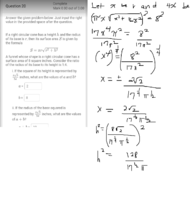# What are the values of a and b?

#### onesun0000

##### Junior Member
I got this question from our quiz wrong I wonder how my teacher got the correct answers shown. I tried solving the first question (on the right side of the image is my solution) but didn't get the right answers. I know the formula for the surface of a right circular cone is $$\displaystyle S=πr(r+\sqrt {r^2+h^2})$$ but I still followed the given formula for the sake of trying to obtain what my teacher got.#### Jomo

##### Elite Member
What you did is fine but not complete. Since your answer is not in the correct form that means you want to multiply it by 1 to make it look like the form you want (If you multiply your answer by other than 1 it will obviously chance its value).

So you need to decide what it is about the form you have that makes it different from the required form. If you can't see this then write back and we'll give you another hint.